# Mechanical Energy | conservation of Mechanical energy – derivation Class 11

• 2
•
• 1
•
• 2
•
•
•
5
Shares

Here we will know what is mechanical energy of a system under study , when we can conserve the mechanical energy of system ? We will derive it.

## Mechanical Energy

### Introduction to mechanical energy

In classical mechanics , we deal with macroscopic systems , velocities and forces operating on them. Mechanical energy of a macroscopic system is the sum of energy of the system due to its motion and configuration of system i.e it’s the sum of potential energy and kinetic energy of a system . The principle of conservation of Mechanical energy States that the mechanical energy of an isolated system which is acted upon only by conservative forces remains constant. We’d already discussed the analysis of conservative force on an system.Note

In situations which we deal in real life there are non conservative forces like friction are present but sometimes these are very negligible in comparison to the total energy and energy lost due to this will be very minute in amout , in such cases Energy conservation holds to a very good approximation.

When non conservative forces like friction acts on system then there is a loss in mechanical energy in the form of heat energy, sound energy which we’d observed in our daily life.

We’d also observed that if in a system friction acts then there is a rise in temperature of any system there is a relation  the mechanical energy lost equals  the change in the temperature of the system which was given discovered by James Prescott joule.

#### Kinetic Energy

Kinetic energy of a object is the energy due to motion of a object and the kinetic energy of a system is the sum of the kinetic energies of individual objects in motion in the system. Because energy is the quantity having magnitude and there is no meaning of direction of energy as we say how much energy a body contains not in which direction energy is in body, hence it’s a scalar quantity.

Qualitatively, the work done by the total forces equals the energy gained by the object and this energy is kinetic energy it comes out to be equal to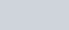Where v is the speed of the all object. Must see the derivation of kinetic energy.

#### Potential energy

If a system is being acted upon by conservative forces then there is energy defined due to configuration of system as potential energy , corresponding to each conservative force potential energy is defined seperately. Must see how potential energy is defined.

## Principle of Conservation of Mechanical energy

The principle of conservation of Mechanical energy States that

the total mechanical energy of an isolated system having no external forces and all internal forces are conservative forces , remains constant .

Mechanical energy is sum of kinetic energy and potential energy i.e.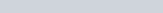This sum of potential energy and kinetic energy remains constant individually potential energy and kinetic energy can change but the summation of this remains constant.

### Derivation of conservation of Mechanical energy

#### Basic Qualitative derivation

We know from Work-Energy theorem that the work done by total forces whether internal or external forces equals the change in kinetic energy of system.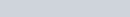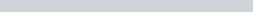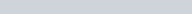Internal forces classified as conservative and non conservative.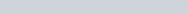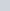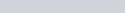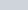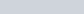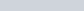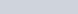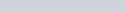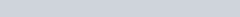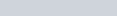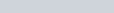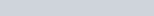The above expression states that the sum of initial kinetic energy and initial potential energy equals to the sum of final kinetic energy and final potential energy, means
That the initial mechanical energy equals to the final mechanical energy hence the mechanical energy is conserved when work done by non conservative forces and external forces equals zero.

#### Derivation Using Calculus

Let’s take a system acted upon by conservative forces only.

(1)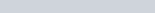(2)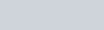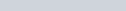(3)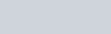From equation (2) and (3)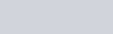(4)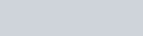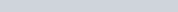(5)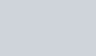Also

(6)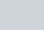Using equation (5) and (6) equation (4) becomes(7)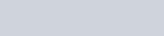The Quantity inside brackets in equation (7) is known as mechanical energy of a system and is the sum of kinetic energy and potential energy. As the derivative of Mechanical energy w.r.t time is zero this implies that the mechanical energy isn’t changing w.r.t time, hence it’s conserved and constant, provided non conservative forces and external forces doesn’t work on system as stated earlier while doing derivation.

Equation (7) can also be seen as a derivation for kinetic energy formula since we derived it without using the concept of kinetic energy.